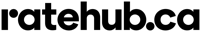## Property transfer tax calculator

As of writing, the property transfer tax (PTT) calculator above does not include the premium rate of 2% for properties with assessed values greater than \$3,000,000.  To calculate your total PTT value, calculate 2% of any amount greater than \$3,000,000 (e.g. if your assessed value is \$3,645,000, you would compute 2% of \$645,000 = \$12,900) and add this amount to the tax calculated above (e.g. a property of \$3,645,000 would have a total PTT of \$87,350 + \$12,900 = \$100,250.  Do not hesitate to reach out to me if you have any questions about this calculation.

## CMHC insurance calculator

Mortgage calculator by